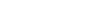# How to convert MQ-9 value to CO ppm concentration?

I have a few questions on how to use the MQ-9 sensor. I’ll refer to the MQ-9 datasheet.

The python sample code is just plain wrong. The arduino sample is at least trying to do the right thing. But it has a few problems. Looks like it divides by 1024 instead of 4096 for a 12 bit ADC. Then it divides by a magic number 3.78, which maybe is supposed to take into account both the load resistance RL and the sensor Ro value at the same time, but it doesn’t explain it. Then it stops with the Rs/Ro ratio and calls it a concentration which it is not. It skips the part about converting the resistance ratio into a gas concentration.

So to do it right, we need to know a few things. What is the value of the load resistance RL? It looks like this is supposed to be set with a potentiometer at manufacturing time to 10 kohm, is that correct? Are they set this way at the NCD factory?

The datasheet does not give a value for the MQ9 Ro resistance. It is defined as “the resistance of sensor in 1000ppm LPG”. We don’t have the capability to measure this. What is the nominal value of Ro that could be applied to all MQ9 sensors? Or does Ro vary so widely from sensor to sensor that you can’t use a nominal value?

I see a jumper to select between 1.5V and 5V. I assume this is for the sensor heater voltage VH. But I think this is also changing the sensor Vc loop voltage too, which surprised me. Is this jumper supposed to change both at the same time? Are these sensor pins A and H tied together? This matters, because the equation to find Rs from the measured voltage depends on the Vc loop voltage.

The datasheet figure 1 is a chart showing the relationship between the ratio Rs/Ro and the gas concentrations in ppm. Is this chart valid for CO when VH =1.5V? The datasheet says to use VH = 1.5V to detect CO, but it kind of looks like the chart would be for VH=5V because the same chart includes other gases too.